# untitled lambda calculus writing system

this system is a non-linear decorative writing system for representing functions in the lambda calculus. this article assumes a cursory knowledge of what lambda calculus is and why it's cool.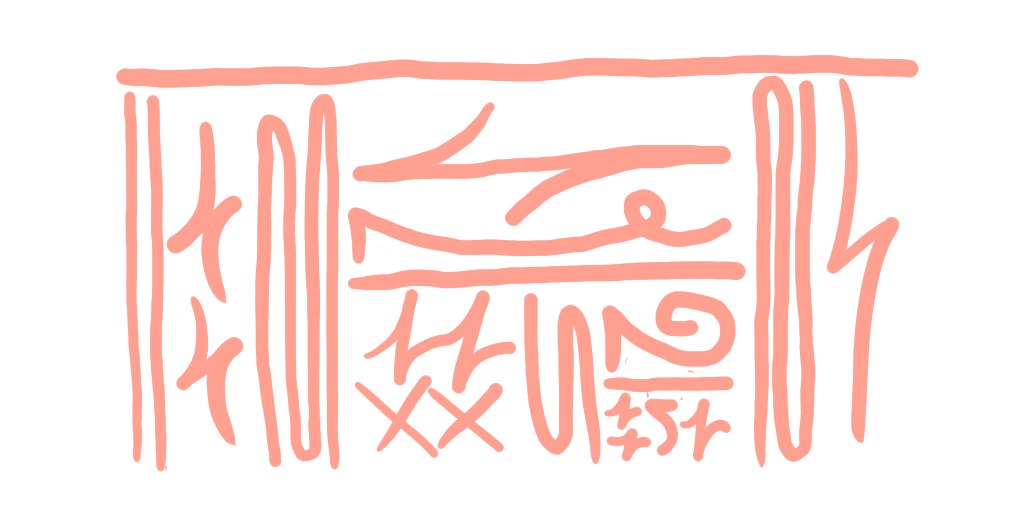the basic idea of a non-linear writing system led me to a few obvious ideas. function application and lambdas could be represented by the structure of the writing, and individual atomic variables could be represented as symbols that fit into the structure. what symbols should i use?

## a quick detour into de bruijn indexing

in most lambda calculus seen in math papers, lambda arguments are bound to names, usually using singular english letters. this is great for understanding how things work: for example, we may have a function that takes arguments f and n and applies f to n twice. since all things look (and are) the same in pure lambda calculus, names guide us to what roles variables take in a program. but technically speaking, we don't need variable names at all! function arguments can be referred to by how many layers of lambdas deep the variable is from the lambda that takes it.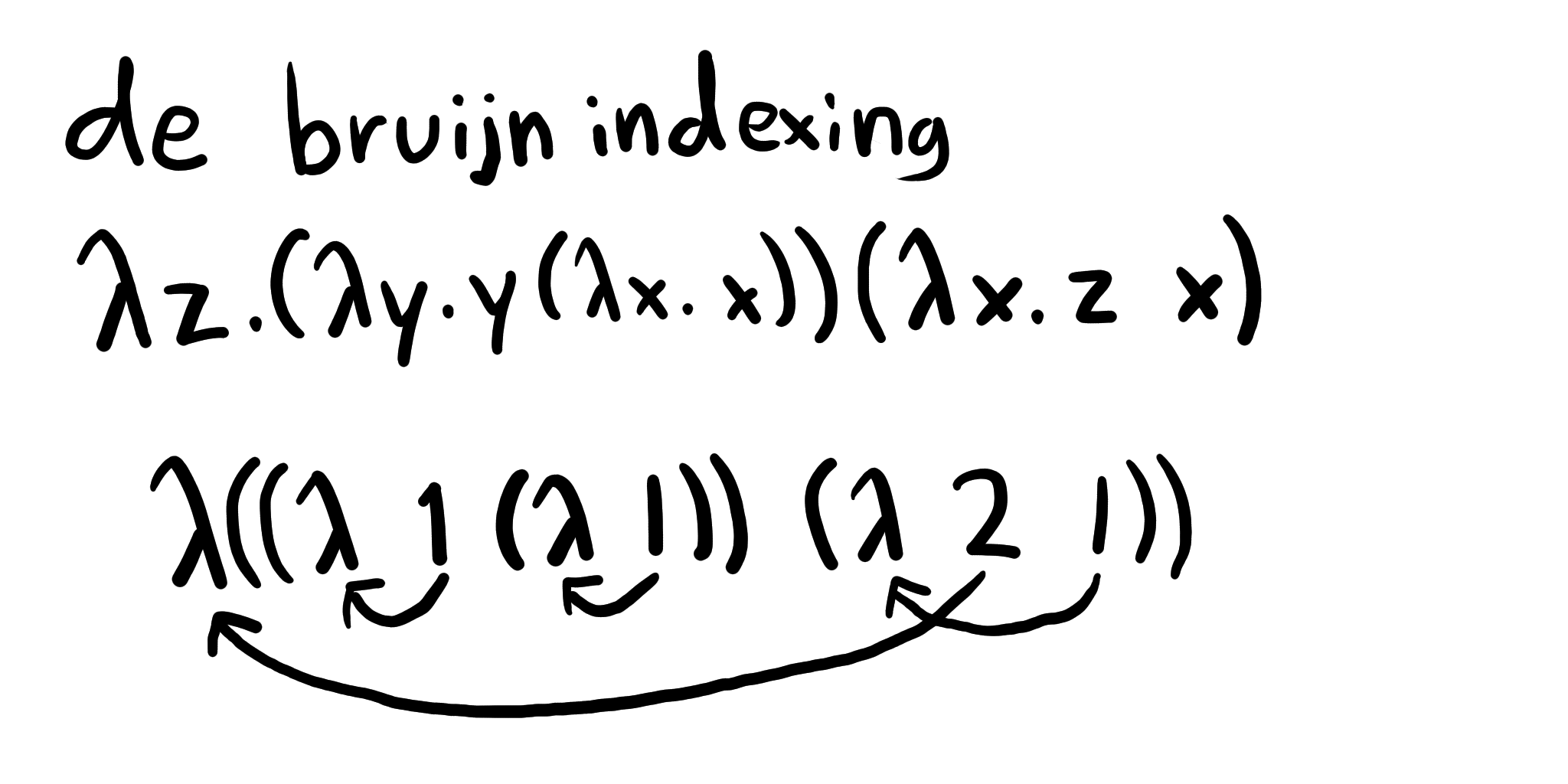this method of representing lambdas isn't very practical for understanding what they do at a glance, but it gives us some nice benefits. for one, each form of a lambda has exactly one representation: both λyy and λxx are represented as λ 1. 1

## the symbols

the system uses base 3 numbering (starting at 1) to represent our symbols. this base number is small but not generally too cumbersome, and the low number of symbols makes them easier to distinguish.

we can represent numbers both vertically and horizontally. each digit can be stretched arbitrarily to fit its needed area. multiple digits are stacked or placed side by side, depending on the orientation.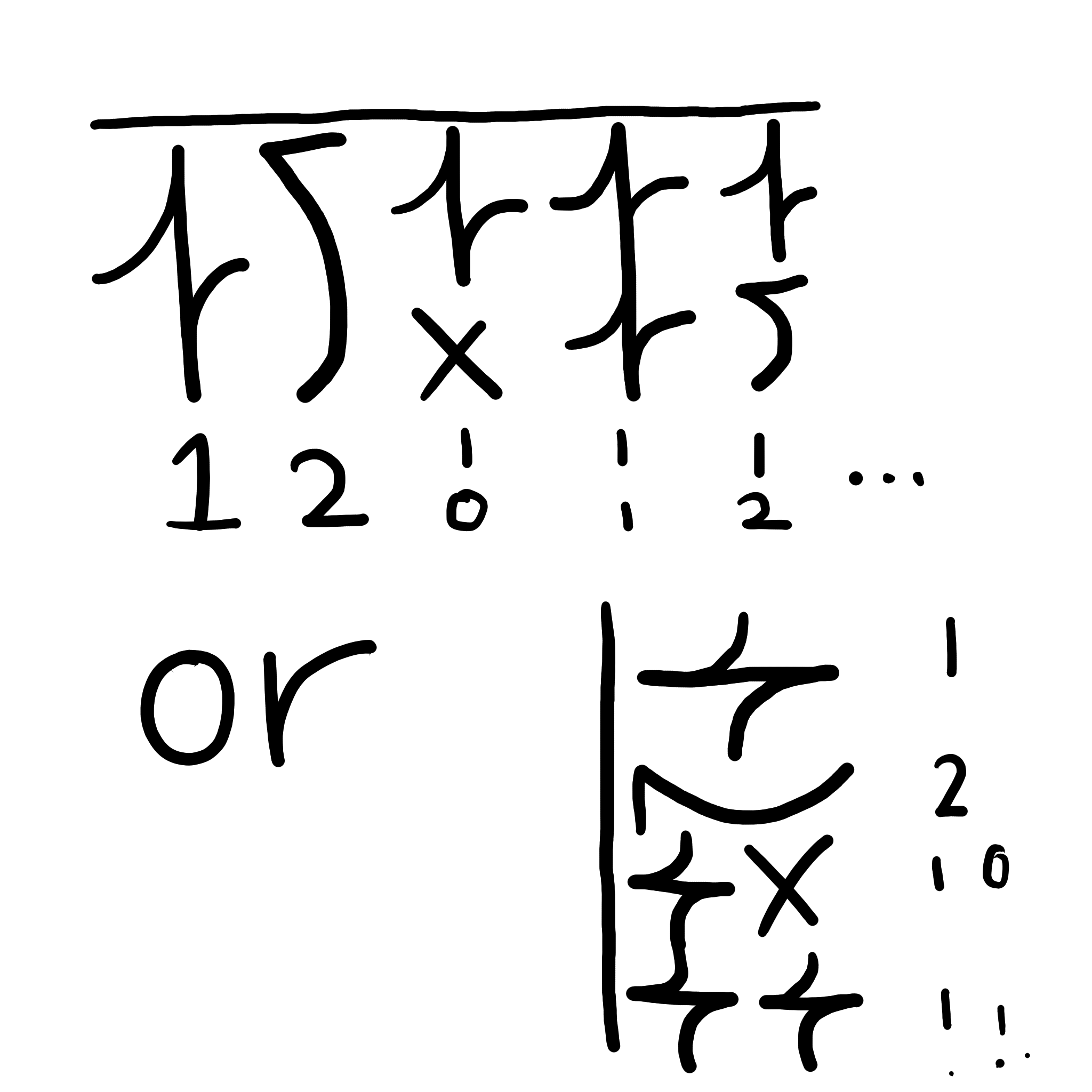## application

in a function application, each argument is placed left-to-right under a horizontal line, or top-to-bottom to the right of a vertical one. when an application is nested inside of another one, the "direction" of the block is switched—a vertical one is placed inside of a horizontal one, and vice versa.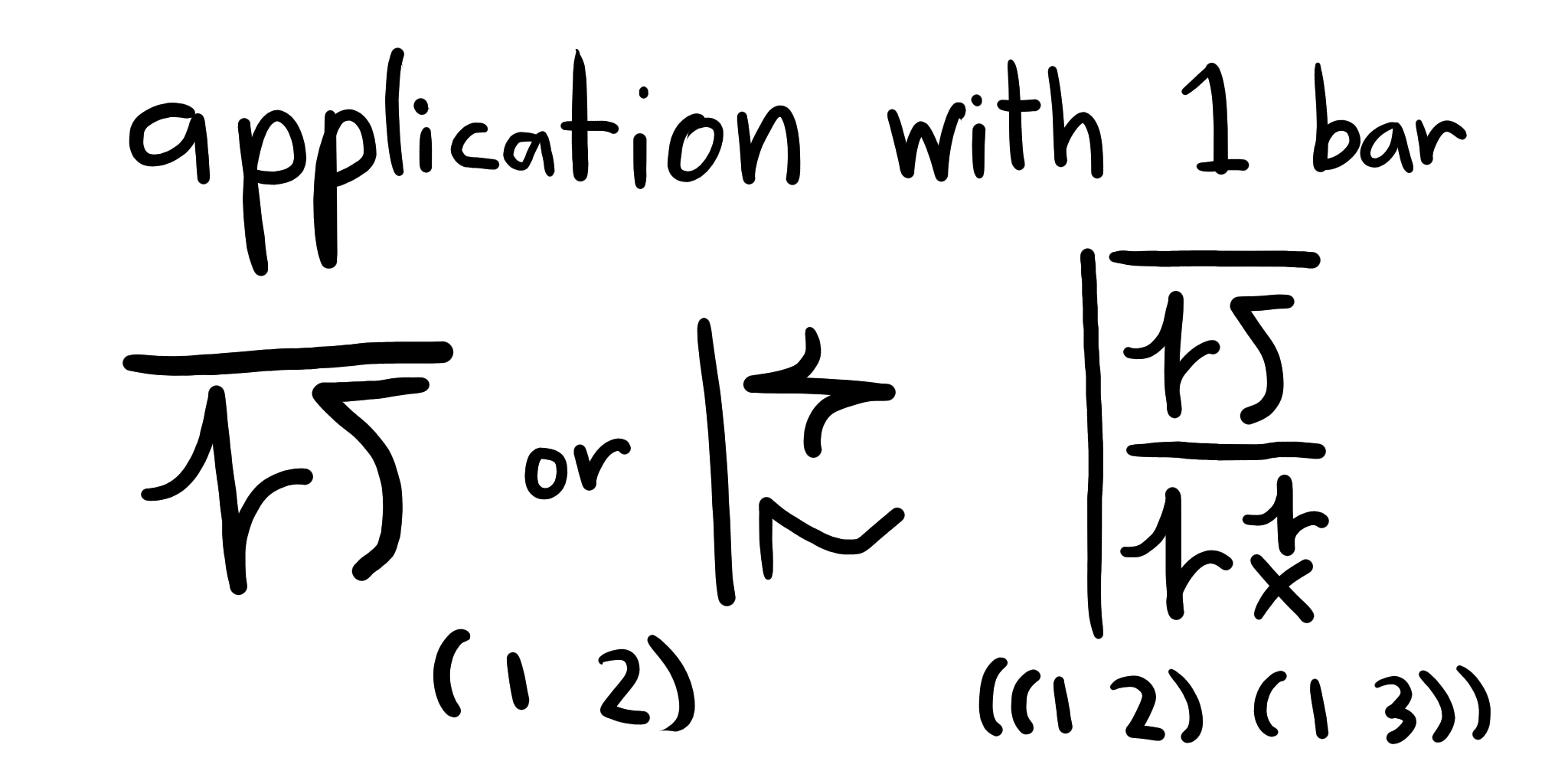## lambdas

lambdas look just like function application, except instead of one bar, there are two or more. two bars represent a single lambda; three bars represent a lambda nested inside another lambda; four bars represent three nested lambdas, etc.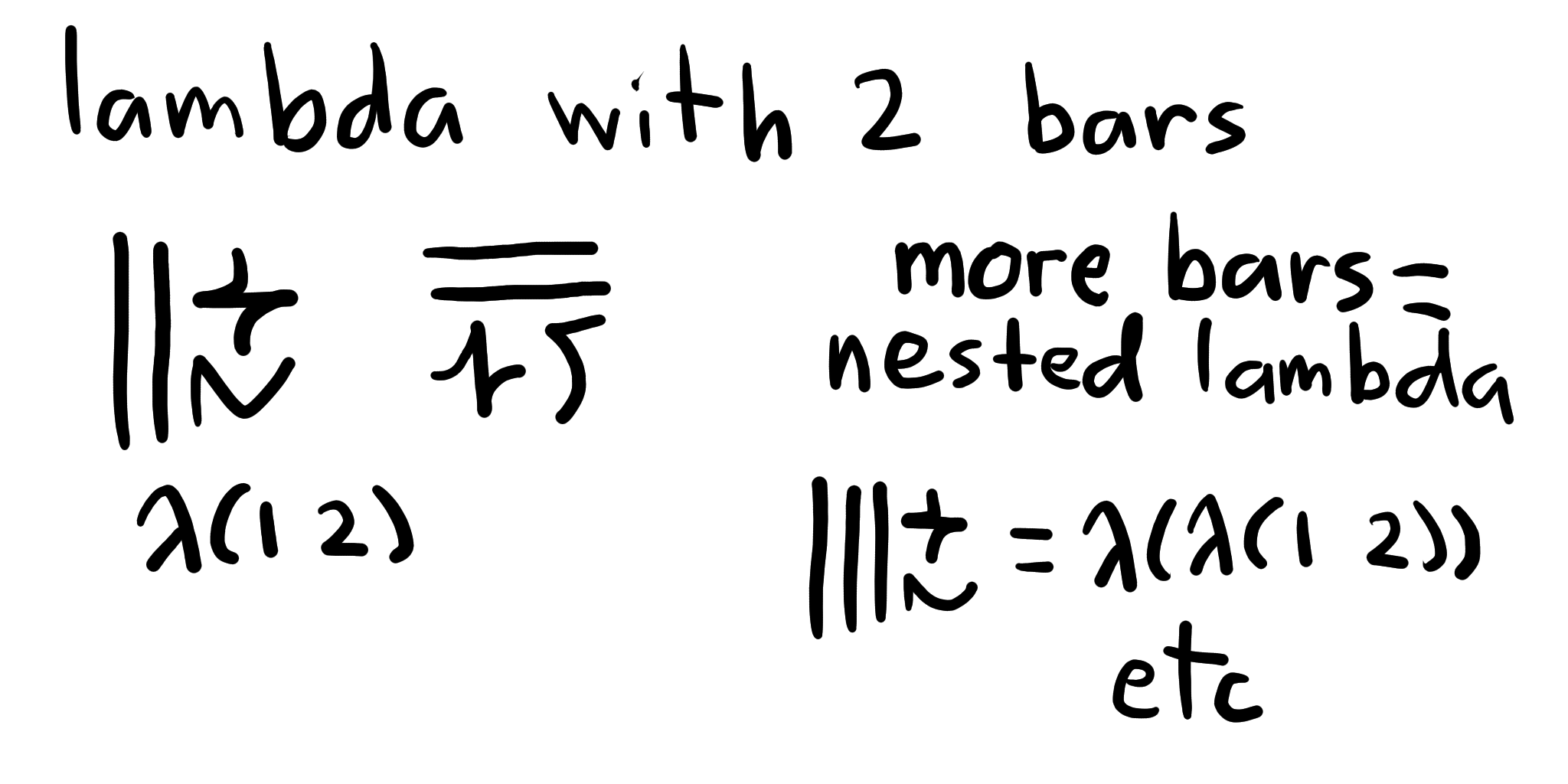## let's go SKIing

let's start with some basic examples: the S, K, and I combinators. it's possible to recreate any lambda using combinations of these simple functions! in order shown, we have I, which returns its only argument; K, which takes two arguments and returns the first; and S, which takes three arguments and applies the combination of its first and third arguments to the combination of its second and third arguments. (it's easier to understand as written.)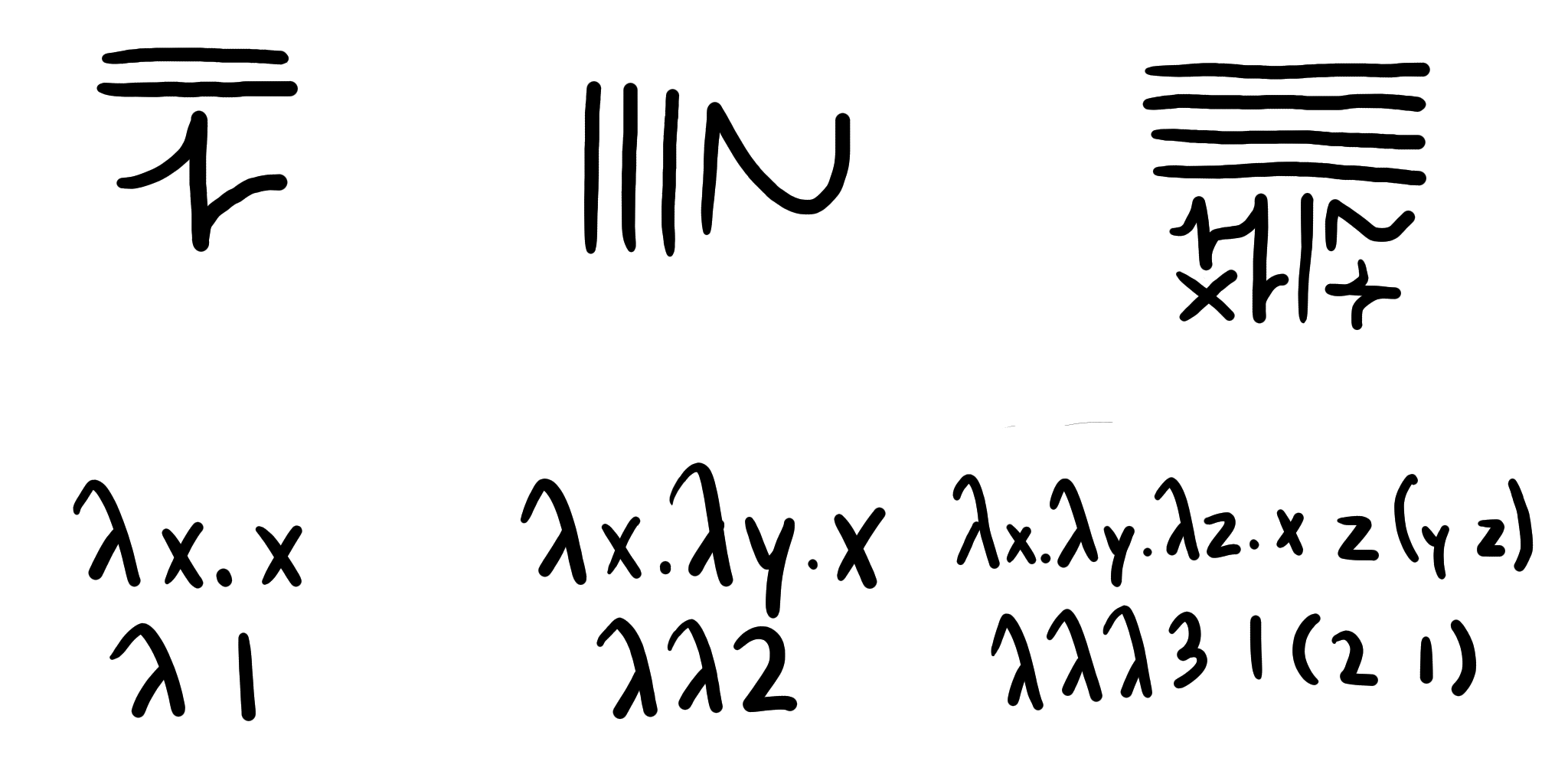## other famous functions

first, we have the Y combinator, which allows recursive functions. second, we have the successor function in the church numerals, which takes an integer encoded as a lambda and returns the integer 1 higher. finally, we have a function that adds two church-numeral numbers. this one kind of looks like a weird man!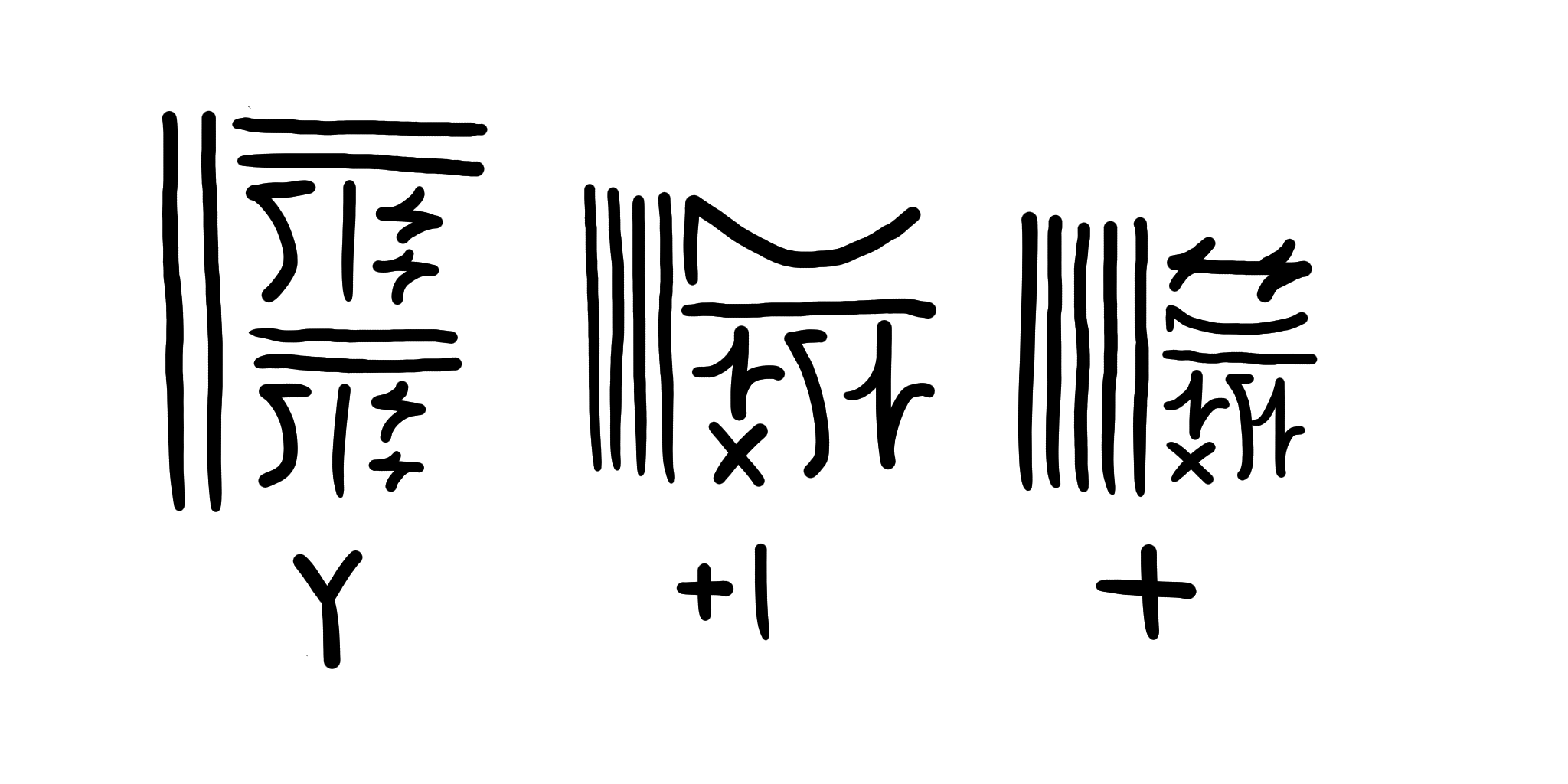1. this negates the need for α-conversion. of course, there are other lambdas that trivially do the same thing but are represented differently.↩︎